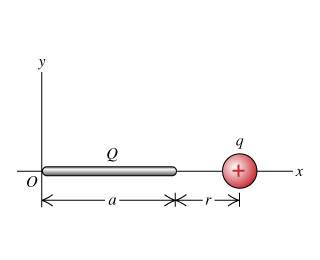# Charge Distribution on x-axis

Hey guys, I'm kind of stuck on how to do this one.Positive charge Q is distributed uniformly along the x-axis from x=0 to x=a. A positive point charge q is located on the positive x-axis at x=a+r, a distance r to the right of the end of Q.

(a) Calculate the x- and y- components of the electric field produced by the charge distribution Q at points on the positive x-axis where x>a.

(b) Calculate the force (magnitude and direction) that the charge distribution Q exerts on q.

(c) Show if r>>a, the magnitude of the force in part (b) is approximately Qq/4pi(epsilon)r^2. Explain why this result is obtained.

Alright, I'm having trouble getting started. First off, I understand that the y components is 0, no need to go there.

I know that since the charge is distributed, I have to use an integral. the Limits of the integral are from (0,a)

But what how exactly is the integral? (I'm going to use k instead of 1/4piepsilon

To my understanding....

E=kq/r^2

So, dE=kdq/r^2

How would I go from there? (if that were correct...)

Thanks!

## Answers and Replies

collinsmark
Homework Helper
Gold Member
Hello btbam91,

I'll attempt to get you started.
To my understanding....

E=kq/r^2
That is correct for a point charge, or for somewhere outside a sphere are shell with spherically symmetrical charge distribution.
So, dE=kdq/r^2

How would I go from there? (if that were correct...)
Now here, be careful about your variable names. The variable 'r' means something very specific to this particular problem. And it isn't the distance from dQ to the test charge. See the figure. The variable 'r' is already taken, so you'll have to create a new variable.

But instead of creating a variable that represents the distance from dQ to the test point, let's just create a variable which specifies where dQ lies on the x-axis.

I'll call this variable 'x'. You can call it something else if you want (you might prefer to call it 'l' to distinguish it from any old point on the x-axis). The variable will vanish when you evaluate the integral anyway, but you need to call it something for now. I'll respecify what x is (or at least what I am calling 'x'): 'x' is the location on the x-axis of the infinitesimal point charge dQ.

The next thing you need to do is come up with an expression for the distance from dQ to the test charge (where the test charge is on the x-axis, at some point greater than a). Create your expression in terms of x, a, and r.

Next break down dQ. dQ is the linear charge distribution times the differential length, dx. (Hint: dQ = λdx.)

You should have enough information to set up your integral now. Integrate over x, where x ranges from 0 to a (a and r can be treated as constants for this integration). The result is that x vanishes and you are left with an expression in terms of a and r.